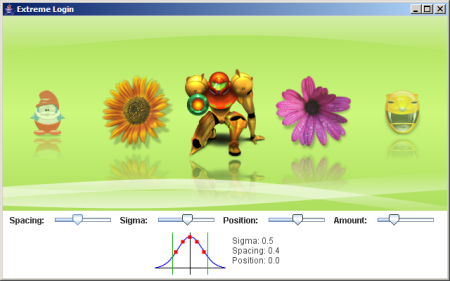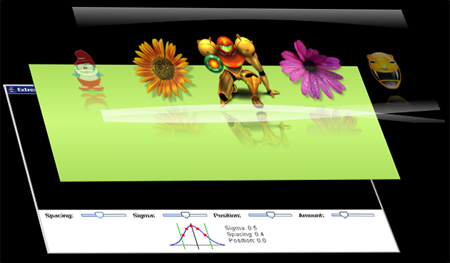## StackLayout

The more demos I write, the more effects I'd like to reuse for other demos. In some cases I'd like to reuse only a part of an effect. In the application I'm writing, I wanted to use the animated curves from Help Your Shelf. In this demo, there is a `CurvePanel` extending `GradientPanel`. My first problem was I wanted to change the gradient and I felt it was awkward to modify the base class rather than just using another implementation. I then added sprites to the demo and I wanted them to be displayed below the curves but on top of the gradient. That's why I decided to decouple every layer and use a `StackLayout` to produce this:To achieve this I simply extracted each graphical element into a separate component: `CurvesPanel`, `GradientPanel` and `AvatarChooser`. With the `StackLayout` it is very easy to stack them in the right order:

```JPanel pane = new JPanel();
pane.setLayout(new StackLayout());

AvatarChooser chooser = new AvatarChooser();
CurvesPanel curves = new CurvesPanel();

```

The API is very simple and let you add a new component either on top or at the bottom of the stack. Here is how the three components are stacked:The code used to create the layout is very simple. As you can see below, it simply changes the Z order of every component and make them take as much space as possible, thus providing the same behavior than the `BorderLayout.CENTER` constraint. Here is the source code:

```import java.awt.Component;
import java.awt.Container;
import java.awt.Dimension;
import java.awt.Insets;
import java.awt.LayoutManager2;
import java.awt.Rectangle;
import java.util.List;
public class StackLayout implements LayoutManager2 {
public static final String BOTTOM = "bottom";
public static final String TOP = "top";
private List components = new LinkedList();
public void addLayoutComponent(Component comp, Object constraints) {
synchronized (comp.getTreeLock()) {
if (BOTTOM.equals(constraints)) {
} else if (TOP.equals(constraints)) {
} else {
}
}
}
public void addLayoutComponent(String name, Component comp) {
}
public void removeLayoutComponent(Component comp) {
synchronized (comp.getTreeLock()) {
components.remove(comp);
}
}
public float getLayoutAlignmentX(Container target) {
return 0.5f;
}
public float getLayoutAlignmentY(Container target) {
return 0.5f;
}
public void invalidateLayout(Container target) {
}
public Dimension preferredLayoutSize(Container parent) {
synchronized (parent.getTreeLock()) {
int width = 0;
int height = 0;
for (Component comp: components) {
Dimension size = comp.getPreferredSize();
width = Math.max(size.width, width);
height = Math.max(size.height, height);
}
Insets insets = parent.getInsets();
width += insets.left + insets.right;
height += insets.top + insets.bottom;
return new Dimension(width, height);
}
}
public Dimension minimumLayoutSize(Container parent) {
synchronized (parent.getTreeLock()) {
int width = 0;
int height = 0;
for (Component comp: components) {
Dimension size = comp.getMinimumSize();
width = Math.max(size.width, width);
height = Math.max(size.height, height);
}
Insets insets = parent.getInsets();
width += insets.left + insets.right;
height += insets.top + insets.bottom;
return new Dimension(width, height);
}
}
public Dimension maximumLayoutSize(Container target) {
return new Dimension(Integer.MAX_VALUE,
Integer.MAX_VALUE);
}
public void layoutContainer(Container parent) {
synchronized (parent.getTreeLock()) {
int width = parent.getWidth();
int height = parent.getHeight();
Rectangle bounds = new Rectangle(0, 0, width, height);
int componentsCount = components.size();
for (int i = 0; i
If you want to develop reusable, non opaque components, this layout can be very handy.
P.S: The white panel is actually used for debugging purpose only.

← Previous post
Next post →

```